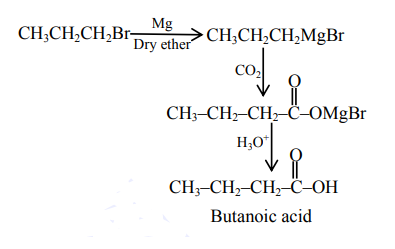# Which one of the following reactions will not yield propionic acid?Question:

Which one of the following reactions will not yield propionic acid?

1. $\mathrm{CH}_{3} \mathrm{CH}_{2} \mathrm{COCH}_{3}+\mathrm{OI}^{-} / \mathrm{H}_{3} \mathrm{O}^{+}$

2. $\mathrm{CH}_{3} \mathrm{CH}_{2} \mathrm{CH}_{3}+\mathrm{KMnO}_{4}$ (Heat), $\mathrm{OH}^{-} / \mathrm{H}_{3} \mathrm{O}^{+}$

3. $\mathrm{CH}_{3} \mathrm{CH}_{2} \mathrm{CCl}_{3}+\mathrm{OH}^{-} / \mathrm{H}_{3} \mathrm{O}^{+}$

4. $\mathrm{CH}_{3} \mathrm{CH}_{2} \mathrm{CH}_{2} \mathrm{Br}+\mathrm{Mg}, \mathrm{CO}_{2}$ dry ether $/ \mathrm{H}_{3} \mathrm{O}^{+}$

Correct Option: , 4

Solution:

All gives propanoic acid as product but option 4 gives butanoic as product